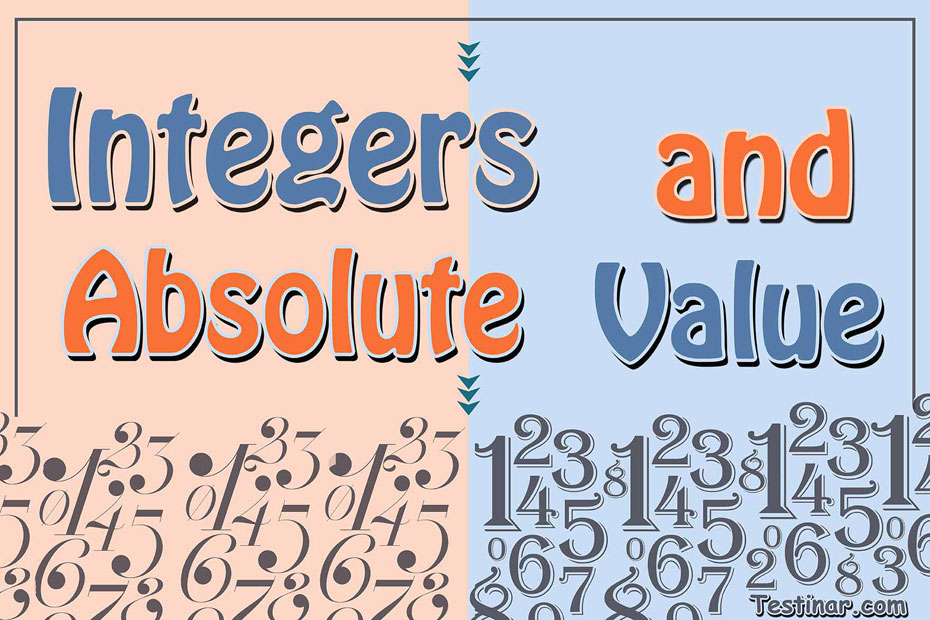## How to Solve Integers and Absolute Value Problems

The term integers represent the meaning “intact” or “whole”. So, you can generally refer an integer as a whole number, except integers can be negative also!

What is an Integer?

An integer is a whole number (not any decimal or fraction numbers) which can be zero, positive or negative numbers. Some examples of integers can be $$7 \ , \ 3 \ , \ 0 \ , \ -5 \ , \ -15,$$ etc. Moreover, we can represent integers by the denotation $$Z$$ which comprises of:
Positive Integers: As the name suggests, any integer that is greater than zero is termed as a positive integer.
Negative Integer:  As from the name, any integer that is less than zero is termed as a negative integer.
Zero: Zero is neither a positive integer or a negative integer. It is just a whole number.
So, we can write $$Z = \{….,-5 \ , \ -4 \ , \ -3 \ , \ -2 \ , \ -1 \ , \ 0 \ , \ 1 \ , \ 2 \ , \ 3 \ , \ 4 \ , \ 5,……\}$$
Also, we can place all integers on a number line where the negative ones are placed on the left of $$“0”$$ and the positive ones on the right. Moreover, we can perform the 4 basic mathematic properties with integers. They are:

• Subtraction
• Multiplication
• Division

We often see that negative integers are always written as $$-5 \ , \ -9$$ and so on. But it is not generally considered necessary to write positive integers like $$+5 \ , \ +9$$ and so on. So, when we write just $$5,$$ we mean $$+5$$.
Another thing to note is, that an absolute value of any integer is always positive. So, $$\lvert -6 \rvert=6$$ and $$\lvert 6 \rvert$$  is also $$6$$.

What is the Absolute Value of an Integer?

If we plot an integer on the number line, then the absolute value of that integer would be its distance from zero. Since distance can’t be negative, therefore the absolute value of any number is always a positive quantity. Absolute value is sometimes even referred to as magnitude. Absolute of the integer $$x$$ is shown as $$\lvert x \rvert$$.
For example, absolute value of $$-4$$ is $$\lvert -4 \rvert= 4$$. This is pretty obvious as when we plot the integer $$4$$ on the number line, we can clearly see that the distance from zero is $$“4”$$.

### Exercises for Integers and Absolute Value

1) $$-14 \ + \ |-8| \ =$$

2) $$-7 \ + \ |0| \ =$$

3) $$0 \ + \ |9| \ =$$

4) $$15 \ + \ |7| \ =$$

5) $$|-15| \ =$$

6) $$|0| \ =$$

7) $$|7| \ =$$

8) $$|-11| \ + \ |4| \ =$$

9) $$|-18| \ + \ |-4| \ =$$

10) $$|4| \ + \ |-6| \ =$$

1) $$-14 \ + \ |-8| \ = \color{red}{-6}$$
2) $$-7 \ + \ |0| \ = \color{red}{-7}$$
3) $$0 \ + \ |9| \ = \color{red}{9}$$
4) $$15 \ + \ |7| \ = \color{red}{22}$$
5) $$|-15| \ = \color{red}{15}$$
6) $$|0| \ = \color{red}{0}$$
7) $$|7| \ = \color{red}{7}$$
8) $$|-11| \ + \ |4| \ = \color{red}{15}$$
9) $$|-18| \ + \ |-4| \ = \color{red}{22}$$
10) $$|4| \ + \ |-6| \ = \color{red}{10}$$

## Integers and Absolute Value Practice Quiz

### Prepare for the PSAT / NMSQT Math Test in 7 Days

$17.99$12.99

### FTCE General Knowledge Math for Beginners 2022

$21.99$13.99

### CLEP College Algebra Exercise Book

$18.99$14.99

### Ace the SSAT Middle LEVEL Math in 30 Days

$20.99$14.99